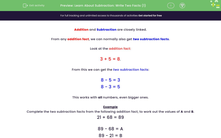# Learn About Subtraction: Write Two Facts (1)

In this worksheet, students learn about two more subtraction facts from each of the given sums.Key stage:  KS 2

Curriculum topic:   Number: Addition and Subtraction

Curriculum subtopic:   Solve Add/Subtract Problems to 1000

Difficulty level:#### Worksheet Overview

From any addition fact, we can normally also get two subtraction facts.

3 + 5 = 8.

From this we can get the two subtraction facts:

8 - 5 = 3

8 - 3 = 5

This works with all numbers, even bigger ones.

Example

Complete the two subtraction facts from the following addition fact, to work out the values of A and B.

21 + 68 = 89

89 - 68 = A

89 - 21 = B

21 + 68 = 89.

From this we can get the two subtraction facts:

89 - 68 = 21

89 - 21 = 68

So we have shown that:

A = 21

B = 68

### What is EdPlace?

We're your National Curriculum aligned online education content provider helping each child succeed in English, maths and science from year 1 to GCSE. With an EdPlace account you’ll be able to track and measure progress, helping each child achieve their best. We build confidence and attainment by personalising each child’s learning at a level that suits them.

Get started